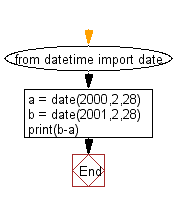﻿ Python: Get days between two dates - w3resource

# Python: Get days between two dates

## Python Datetime: Exercise-18 with Solution

Write a Python program to get days between two dates.

Sample Solution:

Python Code:

``````from datetime import date
a = date(2000,2,28)
b = date(2001,2,28)
print(b-a)
```
```

Sample Output:

```366 days, 0:00:00
```

Flowchart:Python Code Editor: